# Application of Flip Flops

### Registers

Registers are the devices which are meant to store the data. As known, each flip-flop can store a single-bit of information. This means that by cascading n flip-flops, one can store n bits of information. Such an arrangement is called an n-bit register. For example by cascading three D flip-flops as shown in Figure 1, one can store three bits of information (B3, B2 and B1), thus forming a 3-bit buffer register.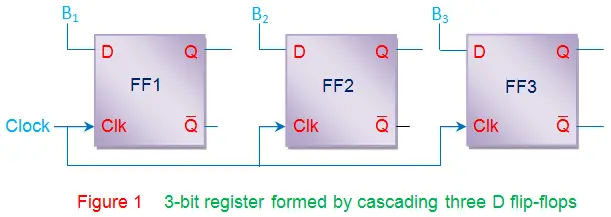The data stored in the registers can be moved stage-wise within the registers and/or in/out of the register by applying clock pulses. Such a register is called shift register. There are various kinds of shift registers depending on the mode of data-shift viz., serial-in serial-out register, serial-in parallel-out register, parallel-in serial-out register, parallel-in parallel-out register. Further depending on the direction of data movement they can be either left-shift and/or right-shift in nature, as shown by Figure 2.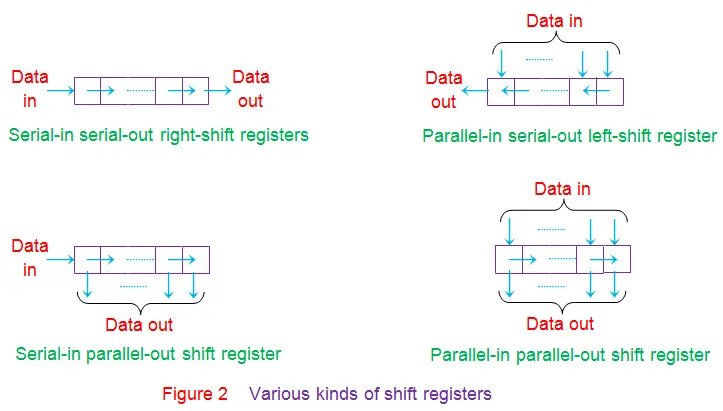### Counters

Counters are the digital circuits which are used to count the number of events. These are nothing but a series of flip-flops (JK or D or T) arranged in a definite manner. A single flip-flop has two states 0 and 1, which means that it can count upto two. Thus one flip-flop forms a 2-bit (or Modulo 2, MOD 2) counter. Similarly to count till 8, one needs to connect 3 (= 23) flip-flops in series as shown in Figure 3. These counters can either be synchronous/asynchronous and/or positive/negative edge-triggered depending on the connections provided at their clock inputs. Moreover by slightly modifying the connections between the flip-flops, various other kinds of counters can be designed viz., up-counter, down-counter, up/down counter, ring counter, johnson counter, etc.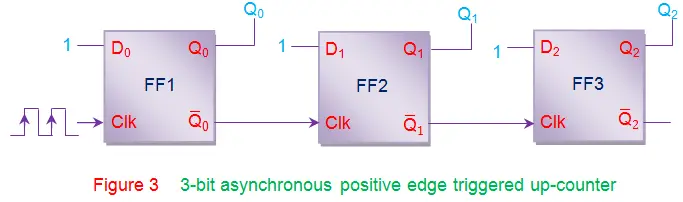### Event Detectors

Event detectors are the circuits which aid in determining the occurrence of a particular event. These devices are required to change their state when an event occurs and should further be held in the same state till that event gets cleared. Flip-flops are well-known to preserve their state until the appearance of a suitable condition at their inputs, which means they can act as event detectors. For example one can use a D flip-flop to detect the event of switching ‘on’ of the light, as shown in Figure 4a. The working of such a circuit is explained in terms of wave forms shown by Figure 4b.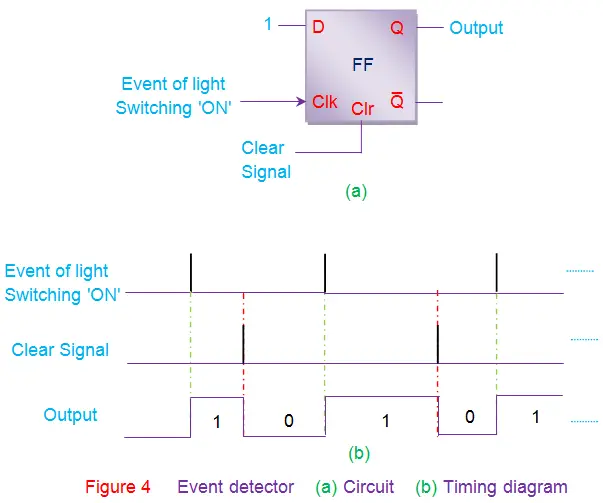### Data Synchronizers

All the outputs of a particular combinational circuit are expected to change their states at the same instant. However sometimes due to the varying gate delays, the outputs of the combinational circuit might change their states at different instants of time (green lines in Figure 5a). This would further cause unexpected behavior resulting in wrong outputs. This can be avoided by using synchronous D flip-flops at the outputs acting as data synchronizers (Figure 5b).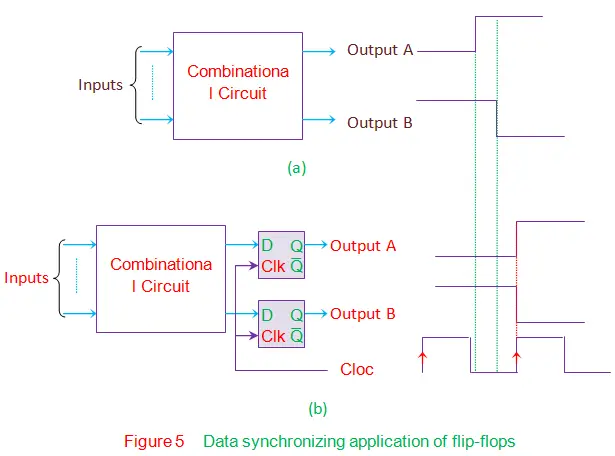In this case, the outputs will be latched by the flip-flops until the clock signal appears. Thus the outputs can change their states only when the positive edge of the clock triggers the flip-flops which in turn causes the state-change in all the outputs at that particular instant of time.

### Frequency Divider

Consider a positive edge triggered JK flip-flop whose inputs are tied-together and driven high, as shown in Figure 6. In this state, the output of JK flip-flop will toggle for each positive-edge of the clock signal (red lines in the figure). From the waveform it is evident that if the input clock period is Tin, then the time period of output waveform Tout is twice of it. Thus one gets fout = fin/2 which implies that input frequency is divided by 2. In other words, after passing through a single flip-flop, the input frequency will be halved. On the same grounds one can conclude that after passing through the n flip-flops, the input frequency will be divided by 2n which results in fout = fin/2n.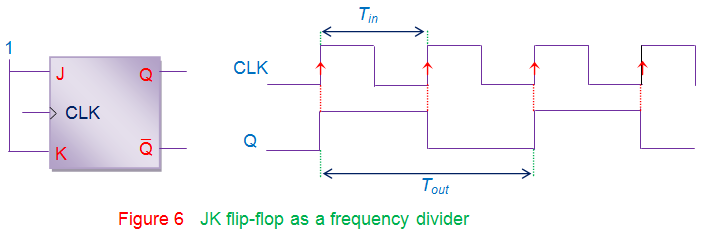Apart from these applications, a few flip-flops have definite uses like

1. D flip-flop can be used to create delay-lines which are used in digital signal processing systems. This application arises readily due to the fact that the output at the synchronous D flip-flop is nothing but the input delayed by one-clock cycle. Thus by cascading n such flip-flops, output can be delayed by n clock cycles which in turn produces the required amount of delay.
2. Generally the mechanical switches used to enter the values into the digital system are prone to bouncing problem where the switch-contacts vibrate while closing/opening the switch. This leads to the variation in the output voltage causing the logical inputs to alternate between 0 and 1. This results in unexpected system behavior which can be avoided by connecting a RS flip-flop between the switch and the digital circuit to act as a switch debouncer.

Want To Learn Faster? 🎓
Get electrical articles delivered to your inbox every week.
No credit card required—it’s 100% free.